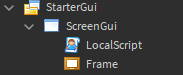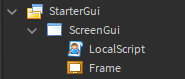# Cloning frames with math.random

Hello, I was experimenting with frames and scripting and I wrote a little script for fun that runs through the client:

``````while true do
local frame = script.Parent.Frame:Clone()
wait(1)
frame.Size = UDim2.new(math.random(0.001, 0.01), 0,math.random(0.001, 0.01), 0)
frame.Position = UDim2.new(math.random(0,1),0,math.random(0,1),0)
end
``````

for some reason it prints but doesn’t do what desired. Every second a new frame is supposed to appear at a random location on the screen, and a random size. Please help2 Likes

You forgot to set the frames parent.

2 Likes

It must clone in same place as original, no?

1 Like

When cloning an object its parent is not set automatically by default.

2 Likes

I tried that. Here’s the new script

``````while true do
wait(1)
local frame = script.Parent.Frame:Clone()
frame.Parent = script.Parent
frame.Size = UDim2.new(math.random(0.001, 0.01), 0,math.random(0.001, 0.01), 0)
frame.Position = UDim2.new(math.random(0,1),0,math.random(0,1),0)
frame.BackgroundColor3 = Color3.new(math.random(0, 255),math.random(0, 255), math.random(0, 255))
frame.ZIndex = math.random(0,100)
end
``````1 Like

Ran it in studio and it works, remember local scripts will only run if you play the game instead of pressing the run button

2 Likes

Am I missing something? I am pressing the “play” instead of run, and I can see the frames not cloning. I don’t know what to do

The frames are also supposed to duplicate, change sizes, colors, and positions.

1 Like

The frames physically dont change position because math.random only returns an integer and you are trying to use it give you a value between 0 and 1

2 Likes

This are lines from my rng game

``````Xpcoor = (math.random(1,10)/10)
Ypcoor = (math.random(1,10)/10)
``````

define them by /10 or /100 and etc.
so this will work
if you use 0.1 or 0.01 this won’t work

1 Like

like this?

``````local frame = script.Parent.Frame:Clone()
while true do
wait(1)
frame.Parent = script.Parent
frame.Size = UDim2.new(math.random(1, 10)/1000, 0,math.random(1, 10)/1000, 0)
frame.Position = UDim2.new(math.random(1,10)/10,0,math.random(1,10)/10,0)
frame.BackgroundColor3 = Color3.new(math.random(1, 255),math.random(1, 255), math.random(1, 255))
frame.ZIndex = (math.random(1,100))
end
``````

Yes, now it’s will work. Test it

1 Like

It works! but it just moves around the same clone over and over again. It’s supposed to make multiple…
EDIT: nevermind, I just moved `local frame = script.Parent.Frame:Clone()` into the while true do statement

Like it’s always on same position or same size or what?

1 Like

This code will work

``````while 1 do
local frame = script.Parent.Frame:Clone()
frame.Size = UDim2.new(math.random(1,1000)/1000, 0,math.random(1,1000)/1000, 0)
frame.Position = UDim2.new(math.random(1,10)/10,0,math.random(1,10)/10,0)
frame.BackgroundColor3 = Color3.fromRGB(math.random(0, 255),math.random(0, 255), math.random(0, 255))
frame.ZIndex = math.random(0,100)
frame.Parent = script.Parent